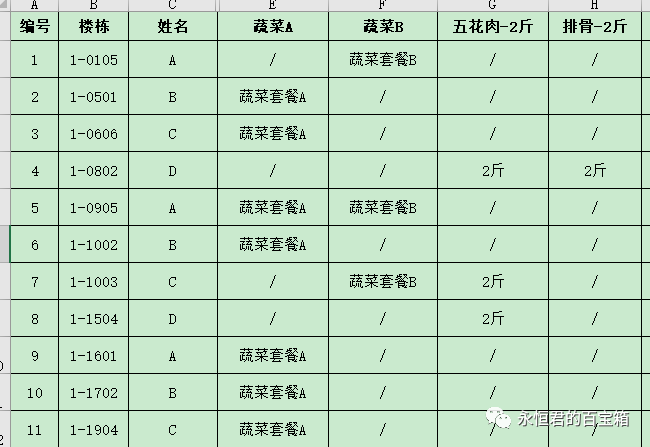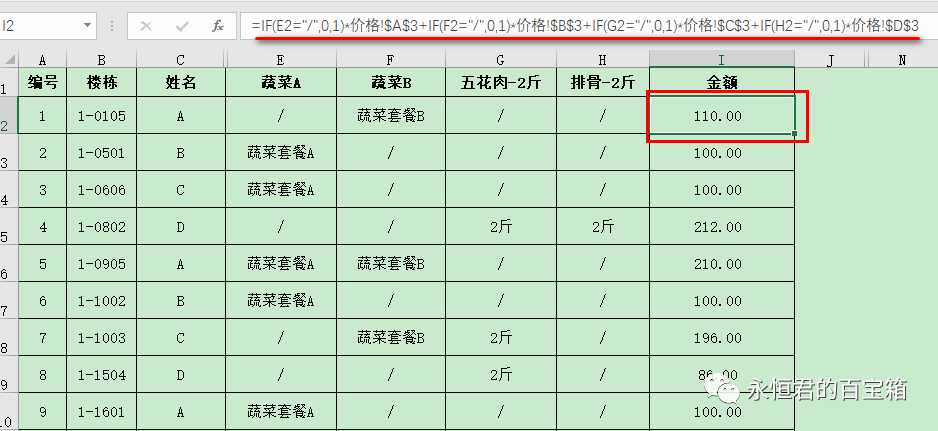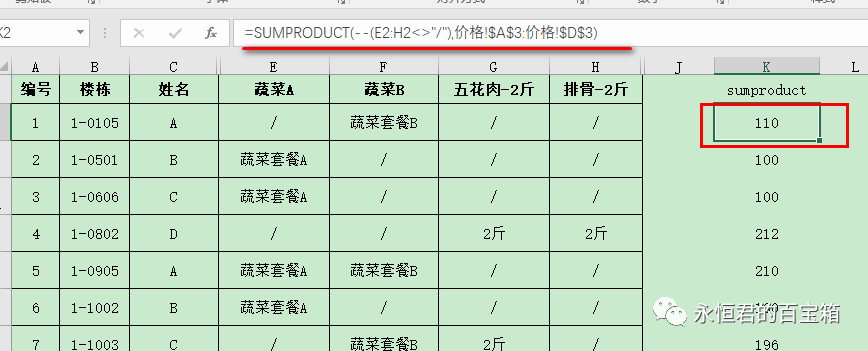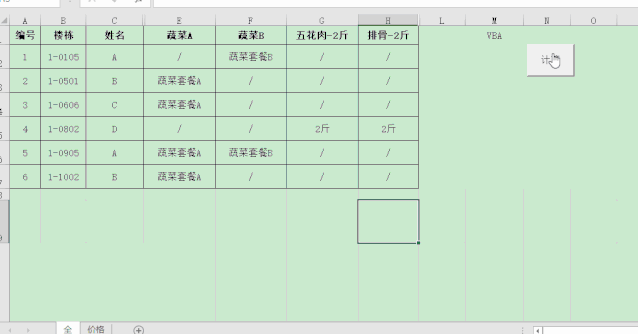﻿ excel如何快速汇总多个类别的综合？ - 永恒君的百宝箱

# excel如何快速汇总多个类别的综合？

1249℃

#### 需求情况#### 解决思路

``````=IF(E2="/",0,1)*价格!\$A\$3+IF(F2="/",0,1)*价格!\$B\$3+IF(G2="/",0,1)*价格!\$C\$3+IF(H2="/",0,1)*价格!\$D\$3
````````````=SUMPRODUCT(--(E2:H2<>"/"),价格!\$A\$3:价格!\$D\$3)
``````SUMPRODUCT()函数的作用就是乘积汇总，--(E2:H2<>"/")表示筛选不等于“/”的项目，标记为1；等于“/”的项目，标记为0；然后再和对应的价格相乘，最后汇总。

``````Sub caijia1()
Range("Q2", "Q10").ClearContents
j = [a2].End(xlDown).Row
For i = 2 To j
Range("M" & i).Value = Evaluate("SUMPRODUCT(--(E" & i & ":H" & i & "<>""/""),价格!\$A\$3:价格!\$D\$3)")
Next
End Sub
``````Excel VBA 实例（24） - 新股（债）中签一键批量查询
Excel VBA 实例（23） - 一键批量提取word表格内容
Excel VBA 实例（22） - 一键筛选其他工作表或工作簿的数据